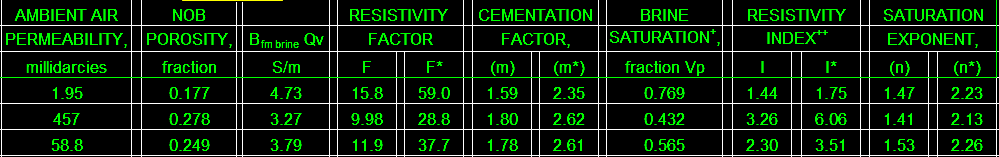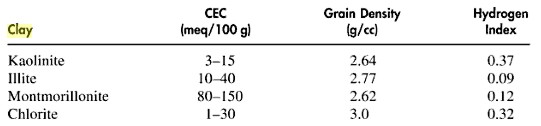WAXMAN-SMITS Saturation (CEC) Method
Another popular method, based on laboratory measured values of cation exchange capacity versus shale content, was developed by Waxman and Smits. It uses the same response equation as in other saturation models, but finds the value for 1/Fsh differently. The method requires a formula for the value of cation exchange capacity, such as the one below:
1: IF PHIe > 0.0
2: THEN CEC = 10 ^ (1.9832 * Vsh - 2.4473)

The above relationship must be derived for each particular area by curve fitting the laboratory data. Some authors have related CEC to porosity in certain areas, but there is no physical reason why this should be true, since specific CEC values depend on shale volume and clay type, and not porosity. The only time this might work is when porosity is strictly a function of shale volume and there are no other mineral variations. Others have tried to relate CEC to some other log data, such as the SP (which of course is a shale indicator), with limited success.  CEC data from laboratory measurements are now routine.

The balance of the equations do not need further modification.
3: RW2 = (RW@FT) * (FT + KT1) / KT5
4: B = 4.6 * (1 - 0.6 exp (-0.77 / RW2))
5: F* = A / (PHIe ^ M*)
6: Qv = CEC * (1 - PHIe) * DENSMA / PHIe
7: Swc = 0.5 * ((- B * Qv * RW2) + ((B * Qv * RW2) ^ 2 + 4 * F* * RW@FT / RESD) ^ 0.5) ^ (2 / N*)
8: OTHERWISE Swc = 1.0

Where:
KT1 = 6.8 for English units
KT1 = 21.5 for Metric units
KT5 = 83.8 for English units
KT5 = 46.5 for Metric units
A = tortuosity exponent (unitless)
B = equivalent conductance of clay cation (mS/m)
CEC = cation exchange capacity of shale (meq/gm)
DENSMA = matrix density (gm/cc or kg/m3)
F* = formation factor (unitless)
FT = formation temperature (degrees Fahrenheit or Celsius)
M* = cementation exponent (unitless)
N* = saturation exponent (unitless)
PHIe = effective porosity (fractional)
Qv = counter ion concentration (meq/gm)
RESD = deep resistivity log reading (ohm-m)
RW2 = water resistivity at 77 degrees Fahrenheit (ohm-m)
RW@FT = water resistivity at formation temperature (ohm-m)
Swc = water saturation from CEC method (fractional)
Vsh = shale volume (fractional)COMMENTS:
When Vsh = 0, then CEC = Qv = 0 and equation reverts to the Archie model.

The product B * Qv is available on modern lab reports of electrical properties, but a relationship between BQv and a well log property such as Vsh is still needed.This set of electrical properties is very detailed but is not sufficient to apply to a log analysis without many more data samples covering a wider spread of reservoir properties.An alternative is to calculate CEC from a clay

mineral analysis based on elemental capture spectroscopy (ECS) log:
9: CEC = Sum(CECi * Vclayi)

Reference:
1. Electrical Conductivities in Oil Bearing Shaly Sands, M. Waxman, L. Smits, SPEJ, June 1968.

Review the references on this method before attempting to use it.

Good CEC data is still hard to come by. CEC measured on core and sample chips often do not correlate well with either effective porosity or shale content, most likely due to the fact that more than one clay mineral is present, each in varying  proportions. Thus a pragmatic fit of CEC to a log derived porosity or shale volume is usually necessary. This field specific approach is commonly applied by those who insist on using the Waxman-Smits approach even when the lack of data does not support its use.

Some analysts use density porosity (PHID), uncorrected for shale, to predict CEC. Some use PHID in the saturation equations instead of PHIe. Others call PHID the “total porosity”, which is wrong, since the standard definition of total porosity is (PHIN + PHID) / 2. These terminology problems stem from shortcuts used in specific areas before sophisticated computer programs made it easy to do better work. Unfortunately, younger analysts learn the tricks of the trade from older analysts who have long forgotten that the shortcut was ever taken.RECOMMENDED PARAMETERS:
In the absense of measured shaly sand electrical properties, use A = 1.00  M* = 2.00  N* = 2.00.NUMERICAL EXAMPLE:
Data for Sand "D"
RESD = 1.0 ohm-
PHIe = 0.11
Vsh = 0.33
A = 0.62
M = 2.15
N = 2.00
RSH = 4.0 ohm-m
RW@FT = 0.015 ohm-m
DENSMA = 2650 kg/m3
FT = 43 degrees Celsius
CEC = 10 ^ (1.9832 * 0.33 - 2.4473) = 0.0161
RW2 = 0.015 * (43 + 21.5) / (83.8 - 37.3) = 0.0208
B = 4.6 * (1 - 0.6 * exp(-0.77 / 0.0208)) = 4.6
F = 0.62 / (0.11 ^ 2.15) = 71.35
Qv = 0.0161 * (1 - 0.11) * 2.650 / 0.11 = 0.3452
Swc = 0.5 * ((-4.6 * 0.3452 * 0.0208) + ((4.6 * 0.3452 * 0.0208)^2+ 4 * 71.35 * 0.015 / 1.0)^0.5)^(2 / 2.0)
= 0.5 * (0.0330 + (0.0011 + 4.281) ^ 0.5) ^ (2 / 2)
Swc = 1.05

If Qv or Vsh were higher the saturation would be lower.

Page Views ---- Since 01 Jan 2015
Copyright 2023 by Accessible Petrophysics Ltd.
CPH Logo, "CPH", "CPH Gold Member", "CPH Platinum Member", "Crain's Rules", "Meta/Log", "Computer-Ready-Math", "Petro/Fusion Scripts" are Trademarks of the Author